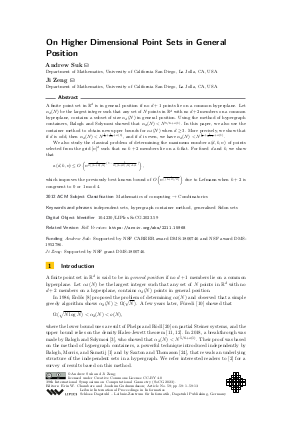Document# On Higher Dimensional Point Sets in General Position

### Authors Andrew Suk, Ji Zeng## File

LIPIcs.SoCG.2023.59.pdf
• Filesize: 0.64 MB
• 13 pages

## Cite As

Andrew Suk and Ji Zeng. On Higher Dimensional Point Sets in General Position. In 39th International Symposium on Computational Geometry (SoCG 2023). Leibniz International Proceedings in Informatics (LIPIcs), Volume 258, pp. 59:1-59:13, Schloss Dagstuhl - Leibniz-Zentrum für Informatik (2023)
https://doi.org/10.4230/LIPIcs.SoCG.2023.59

## Abstract

A finite point set in ℝ^d is in general position if no d + 1 points lie on a common hyperplane. Let α_d(N) be the largest integer such that any set of N points in ℝ^d with no d + 2 members on a common hyperplane, contains a subset of size α_d(N) in general position. Using the method of hypergraph containers, Balogh and Solymosi showed that α₂(N) < N^{5/6 + o(1)}. In this paper, we also use the container method to obtain new upper bounds for α_d(N) when d ≥ 3. More precisely, we show that if d is odd, then α_d(N) < N^{1/2 + 1/(2d) + o(1)}, and if d is even, we have α_d(N) < N^{1/2 + 1/(d-1) + o(1)}. We also study the classical problem of determining the maximum number a(d,k,n) of points selected from the grid [n]^d such that no k + 2 members lie on a k-flat. For fixed d and k, we show that a(d,k,n)≤ O(n^{d/{2⌊(k+2)/4⌋}(1- 1/{2⌊(k+2)/4⌋d+1})}), which improves the previously best known bound of O(n^{d/⌊(k + 2)/2⌋}) due to Lefmann when k+2 is congruent to 0 or 1 mod 4.

## Subject Classification

##### ACM Subject Classification
• Mathematics of computing → Combinatorics
##### Keywords
• independent sets
• hypergraph container method
• generalised Sidon sets

## Metrics

• Access Statistics
• Total Accesses (updated on a weekly basis)
0

## References

1. József Balogh, Robert Morris, and Wojciech Samotij. Independent sets in hypergraphs. Journal of the American Mathematical Society, 28(3):669-709, 2015.2. József Balogh, Robert Morris, and Wojciech Samotij. The method of hypergraph containers. In Proceedings of the International Congress of Mathematicians: Rio de Janeiro 2018, pages 3059-3092. World Scientific, 2018.3. József Balogh and József Solymosi. On the number of points in general position in the plane. Discrete Analysis, 16:20pp, 2018.4. Peter Braß and Christian Knauer. On counting point-hyperplane incidences. Computational Geometry, 25(1-2):13-20, 2003.5. Jean Cardinal, Csaba D Tóth, and David R Wood. General position subsets and independent hyperplanes in d-space. Journal of geometry, 108:33-43, 2017.6. Javier Cilleruelo and Craig Timmons. k-fold Sidon sets. Electronic Journal of Combinatorics, 21(4):P4-12, 2014.7. Henry E Dudeney. Amusements in Mathematics. Nelson, London, 1917.8. Paul Erdös. On some metric and combinatorial geometric problems. Discrete Mathematics, 60:147-153, 1986.9. Achim Flammenkamp. Progress in the no-three-in-line problem, ii. Journal of Combinatorial Theory, Series A, 81(1):108-113, 1998.10. Zoltán Füred. Maximal independent subsets in Steiner systems and in planar sets. SIAM Journal on Discrete Mathematics, 4(2):196-199, 1991.11. H Furstenberg and Y Katznelson. A density version of the Hales-Jewett theorem for k = 3. Discrete Mathematics, 75(1-3):227-241, 1989.12. Hillel Furstenberg and Yitzhak Katznelson. A density version of the Hales-Jewett theorem. Journal d’Analyse Mathématique, 57(1):64-119, 1991.13. Richard R Hall, Terence H Jackson, Anthony Sudbery, and Ken Wild. Some advances in the no-three-in-line problem. Journal of Combinatorial Theory, Series A, 18(3):336-341, 1975.14. Xing De Jia. On finite Sidon sequences. Journal of number theory, 44(1):84-92, 1993.15. Felix Lazebnik and Jacques Verstraëte. On hypergraphs of girth five. Electronic Journal of Combinatorics, 10(1):R25, 2003.16. Hanno Lefmann. No 𝓁 grid-points in spaces of small dimension. In Algorithmic Aspects in Information and Management: 4th International Conference, AAIM 2008, Shanghai, China, June 23-25, 2008. Proceedings 4, pages 259-270. Springer, 2008.17. Hanno Lefmann. Extensions of the no-three-in-line problem. preprint, 2012. URL: www.tu-chemnitz.de/informatik/ThIS/downloads/publications/lefmann_no_three_submitted.pdf.
18. Luka Milićević. Sets in almost general position. Combinatorics, Probability and Computing, 26(5):720-745, 2017.19. Kevin O'Bryant. A complete annotated bibliography of work related to sidon sequences. Electronic Journal of Combinatorics, pages 39-p, 2004.20. Kevin T Phelps and Vojtech Rödl. Steiner triple systems with minimum independence number. Ars combinatoria, 21:167-172, 1986.21. Attila Pór and David R Wood. No-three-in-line-in-3D. Algorithmica, 47(4):481-488, 2007.22. Klaus F Roth. On a problem of Heilbronn. Journal of the London Mathematical Society, 1(3):198-204, 1951.23. Imre Z Ruzsa. Solving a linear equation in a set of integers I. Acta Arithmetica, 65(3):259-282, 1993.24. David Saxton and Andrew Thomason. Hypergraph containers. Inventiones mathematicae, 201(3):925-992, 2015.25. Benny Sudakov and István Tomon. Evasive sets, covering by subspaces, and point-hyperplane incidences. arXiv preprint arXiv:2207.13077, 2022.X

Feedback for Dagstuhl Publishing